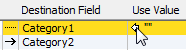## MoneyWorks Manual

Edit

### Using Calculations in Imports

MoneyWorks allows you to massage the data being imported. This means that if the data is incomplete, or doesn’t quite have the right fields, it might be possible to fix this as part of the import process.

To specify a calculation:Double-click on the value to open the Use Value window.
1. Ensure there is no import arrow in the centre column

If there is, click on it to turn it off.

1. Double-click on the value in the right hand column

The Use Value dialog box opens.

You can only do this if no data is being directly imported (i.e. the centre column has no arrow in it).

1. Choose the calculation method

Use Value: If you have a single, known value that you want to apply to all imported records, specify it here;

Work it Out for MeSet this if you want MoneyWorks to provide a value. This is not always available—see Working it Out.

Calculate Value: Set this if you want to calculate a value for the field. This is discussed further below.

Any calculations/values supplied here are normally only applied to new records being imported.

1. If you want this to also apply to the updating of existing records, turn on Set this field when updating existing records as well

Records are only updated if the Update if Exists import option is set (set using the Options button).

1. Click OK

The Use Value window will close, and your value or calculation will be shown in the Value column of the Import Field Order window.

#### Calculations

Calculations can be based on the data on the record being imported, as well as MoneyWorks functions and global variables. The calculation is entered as a normal MoneyWorks expression either direct into the Expression field, or into the Calculation window that opens when you click the Edit button. For information on expressions see Calculations and things.

Note: The fields being imported can be used in an expression by their field name. You can also refer to data in the import file that is not being directly imported by its field number (as shown in the first column). The number is preceded by an underscore, so “_3” would refer to field 3 of the import. For example, if field 4 contains “Money makes the world go around”

```    Left(_4, 3)
```

"Mon", the left 3 characters of field 4

```    left(_4, positionintext(_4, " ")-1)
```

"Money", the first word of field 4

• If you use the * / - operators, MoneyWorks will attempt to coerce the values to numeric. The + operator is for a string concatenation; if you want addition you need to coerce the fields to number by using TextToNum. For example if field 3 contains “10” and field 4 contains “5”
• ```    _3*_4 = 50 (multiplication)
_3 + _4 = 105 (string concatenation)
TextToNum(_3) + TextToNum(_4) = 15 (Addition)
```

1  If you are importing payments or receipts on invoices, you need to use the Import Payments on Invoices command.